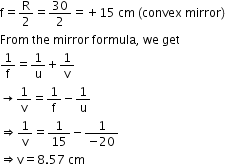3 Tutor System
Starting just at 265/hour

# An object 5 cm is placed at a distance of 20 cm in front of a convex mirror of radius of curvature 30 cm. Find the position, nature and size of the image.

Given:
Object distance, u = -20 cm
Object height, H = 5 cm
Radius of curvature, R = 30 cm
Radius of curvature = 2 × Focal length
$$\Rightarrow$$ R = 2 f
$$\Rightarrow$$ f= $$\frac{R}{2} =\frac{30}{2} =15cm$$The positive value of v indicates that the image is formed behind the mirror.

The positive value of magnification indicates that the image formed is virtual.

m=$$\frac{h'}{h}$$
h' = m × h = 0.428 × 5 = 2.14 cm

The positive value of the image height indicates that the image formed is erect.

Therefore, the image formed is virtual, erect and smaller in size.